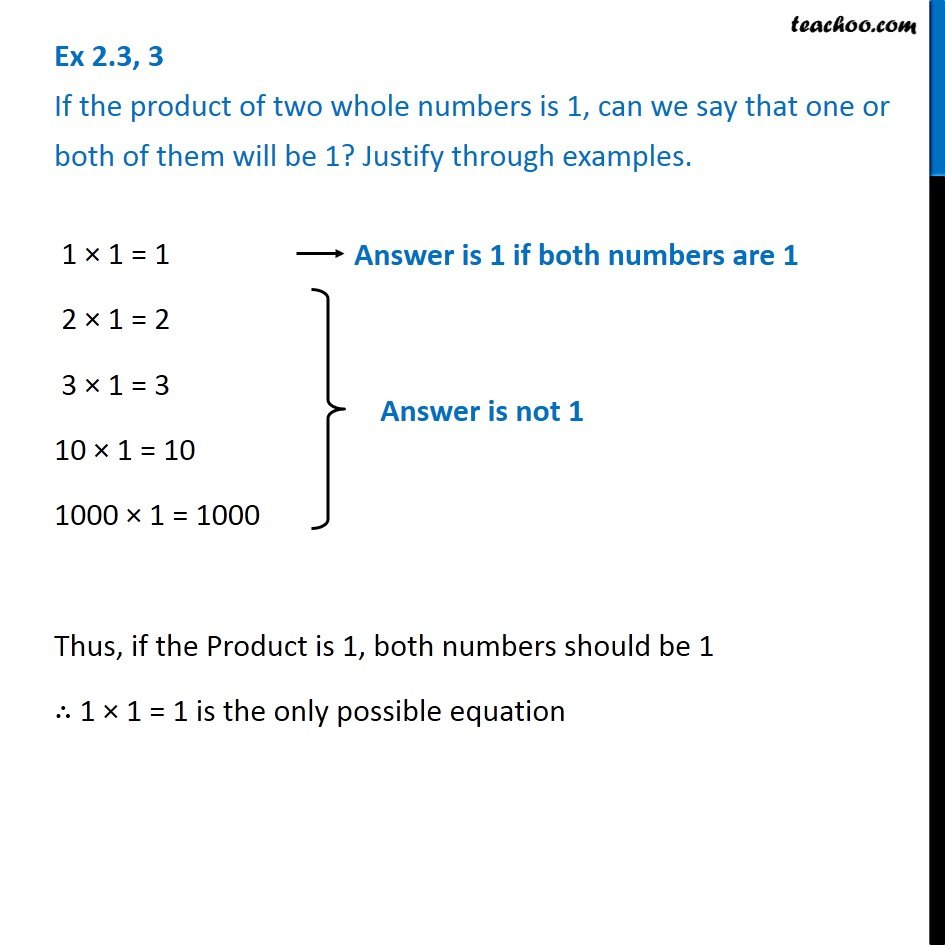Ex 2.3

Chapter 2 Class 6 Whole Numbers
Serial order wiseGet live Maths 1-on-1 Classs - Class 6 to 12

### Transcript

Ex 2.3, 3 If the product of two whole numbers is 1, can we say that one or both of them will be 1? Justify through examples. 1 × 1 = 1 (Answer is 1 if both numbers are 1) 2 × 1 = 2 3 × 1 = 3 10 × 1 = 10 1000 × 1 = 1000 Thus, if the Product is 1, both numbers should be 1 ∴ 1 × 1 = 1 is the only possible equation